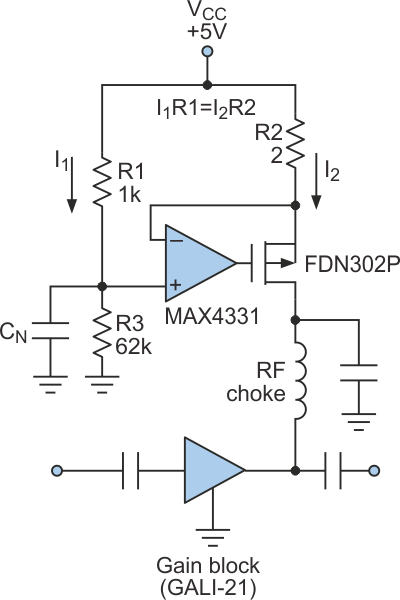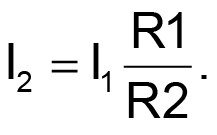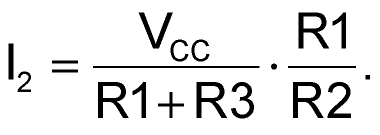# Ensure A Fixed Bias Current For Gain Blocks

## Maxim MAX4331

Ken Yang

Electronic Design

RF gain blocks (amplifiers) are popular because they offer wide bandwidth, low noise, and ease of use. They're designed to operate with a fixed value of supply current because variations in supply current cause variations in the gain, compression point, and other crucial specifications.

Typically, a series resistor sets the supply current to a value based on the known value of dc voltage at the RF choke. That voltage, however, can vary from part to part. For instance, the GALI-21 amplifier (from Mini-Circuits Inc.) specifies a dc output ranging from 3 to 4.1 V.

Therefore, a fixed supply voltage, such as 5 V, allows the supply current to vary ±30%, causing a variation in gain. That behavior is particularly serious in a two-channel system that requires matched gains. To combat this problem, the circuit of the Figure 1 produces a constant and uniform source of current that's independent of part-to-part variations.Figure 1. This simple circuit provides a supply current that is uniform and unaffected by part-to-part variations in the GALI-21 gain block.

Active feedback forces the voltages at the op amp's inverting and non-inverting terminals to be equal. Thus, VR1 = VR2, and:The current ratio is set by resistors R1 and R2, and the gain block's supply current is set by VCC, R1, and R3:In the example circuit, the GALI-21 specifies a supply current of 40 mA. So with VCC = 5 V, choose R2 = 2 Ω (a low resistance), R1 = 1 kΩ, and R3 = 62 kΩ. CN is an optional noise-reduction capacitor.

## Materials on the topic

electronicdesign.com